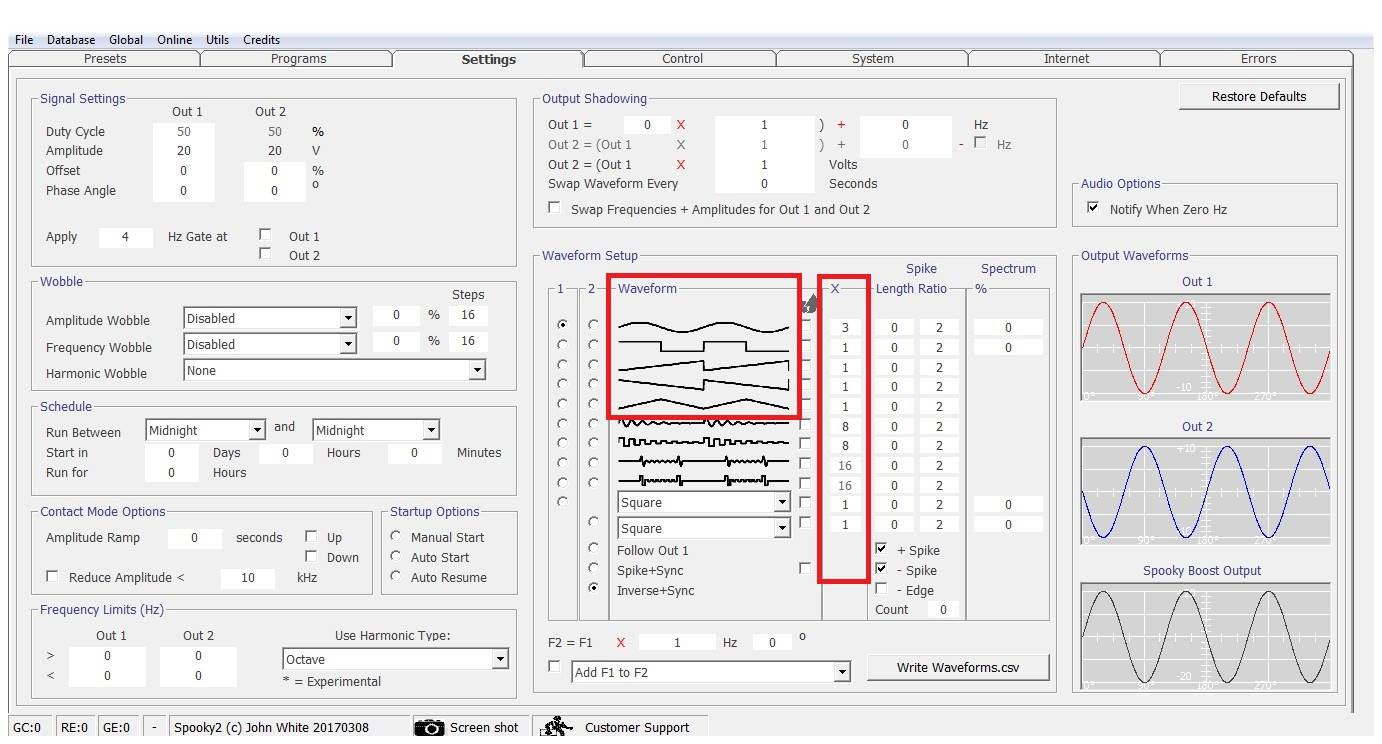# Why the generator frequency is different from the frequency displayed on the computer?

1. The frequency will not be the same if you have a wave cycle multiplier in effect. The other alternative is if you have a frequency limit in place and the frequency has been raised or lowered to another harmonic to fit within the limits. It all depends on what frequency you are looking at on Spooky2 and the settings in use.

When I say it depends on what frequency you are looking at, I mean the original database frequency or what is shown in the Out 1 and Out 2 output panels in the channel control window.

Without knowing exactly what settings you were using, it is hard to determine the exact nature of your observation.

To highlight the wave cycle multiplier closer, if you say are using a Square H Bomb, which has as wave cycle multiplier of 16, in order to achieve the frequency, the generator will play the waveform at 1/16th the rate of the frequency you are targeting.

So if your frequency is 16000 Hz, since the waveform has 16 cycles built in, we will tell the generator to play it at 1000 Hz. 1000 x 16 = 16000 Hz -- the frequency we are after.

http://www.spooky2.com/forums/viewtopic.php?f=6&t=4880

2. There are instances when the frequencies displayed in the generator differs from the Channel Control Display of Outs 1 and 2. This occurs when you are using the following waveforms which have Wave Cycle Multiplier (WCM) [X] of either 11 or 16: Sine Damped Waveform, Square Damped Waveform, Sine H-Bomb and Square H Bomb. To check its accuracy, multiply the WCM (X) number found beside the chosen waveform, either 11 or 16. The generator's frequency should match that of the channel control display for Outs 1 and Out 2.

http://www.spooky2.com/forums/viewtopic.php?f=6&t=5725

3. If you look in the settings tab, find the waveform that is selected for Out 1, then look in the X column to the right of the waveform graph and there is a number different than one, then the generator frequency should be multiplied by that number to indicate the frequency in the program list and Control screen.0 out of 0 found this helpful